# Design a DFA that accepts a string containing 3 a’s and 3 b’s

Problem Statement: Design a Definite Finite Automata for accepting the permutation of Three a’s and Three b’s over the input {a, b}

Input: S = “aaabbb”
Output: Accepted
Explanation:
The input has three a and three b.

Input: S = “abababa”
Output: Accepted
Explanation:
The input has three a and three b.

To design a DFA we need to check the input character by character. These are few steps which should be kept in mind while designing a DFA.

• Think of all the most of the possible input that can be accept (like, in this example, aaabbb, bbbaaa, ababab, abaabb…etc).
• Create a initial transition states.
• Do transition on each input alphabet to other transition state.
• Reach a final state such that in each step the language used was accept(like, in this example, equal number of a and b).

Designing steps:
Step 1: Create a initial state “A” which is indicated by —>.
Step 2: Think of the possible string the can aaabbb or bbbaaa which can occur by transition like,

• Transition of input ‘a’ from state “A” to state “B”
• Transition of input ‘a’ from state “B” to state “C”
• Transition of input ‘a’ from state “C” to state “D”
• Transition of input ‘a’ from state “D” to dead state “Q2”
• Transition of input ‘b’ from state “D” to state “E”
• Transition of input ‘b’ from state “E” to state “F”
• Transition of input ‘b’ from state “F” to state “G”
• Transition of input ‘b’ from state “G” to dead state “Q2”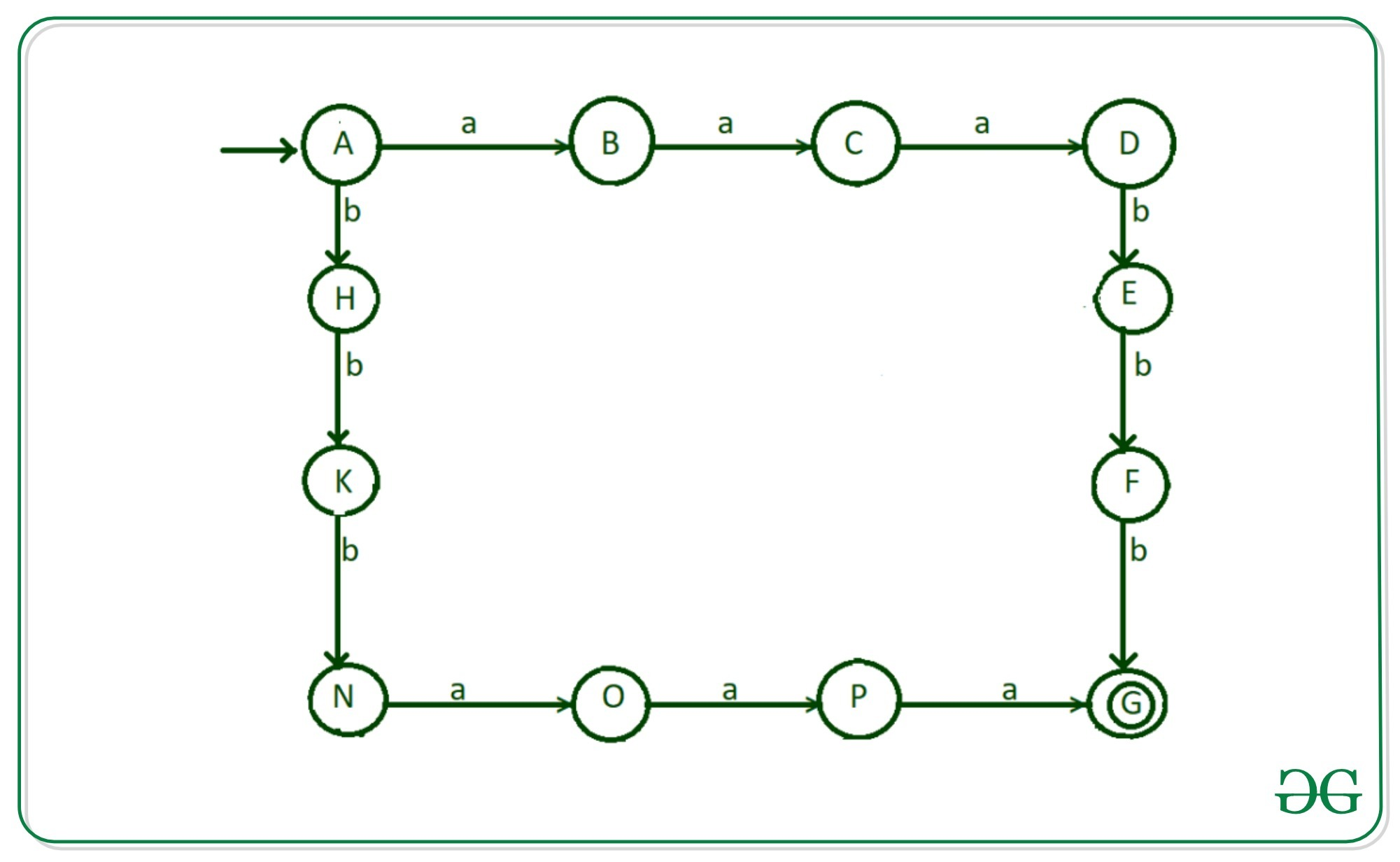Step 3: Now work on possibility like b’s comes after two a’s for example aababb, aabbab.

• Transition of ‘b’ from state “C” to state “J”
• Transition of ‘a’ from state “J” to state “E”
• Transition of ‘b’ from state “J” to state “M”
• Transition of ‘a’ from state “M” to state “F”
• Transition of ‘b’ from state “M” to state “P”

The transition are shown in diagram.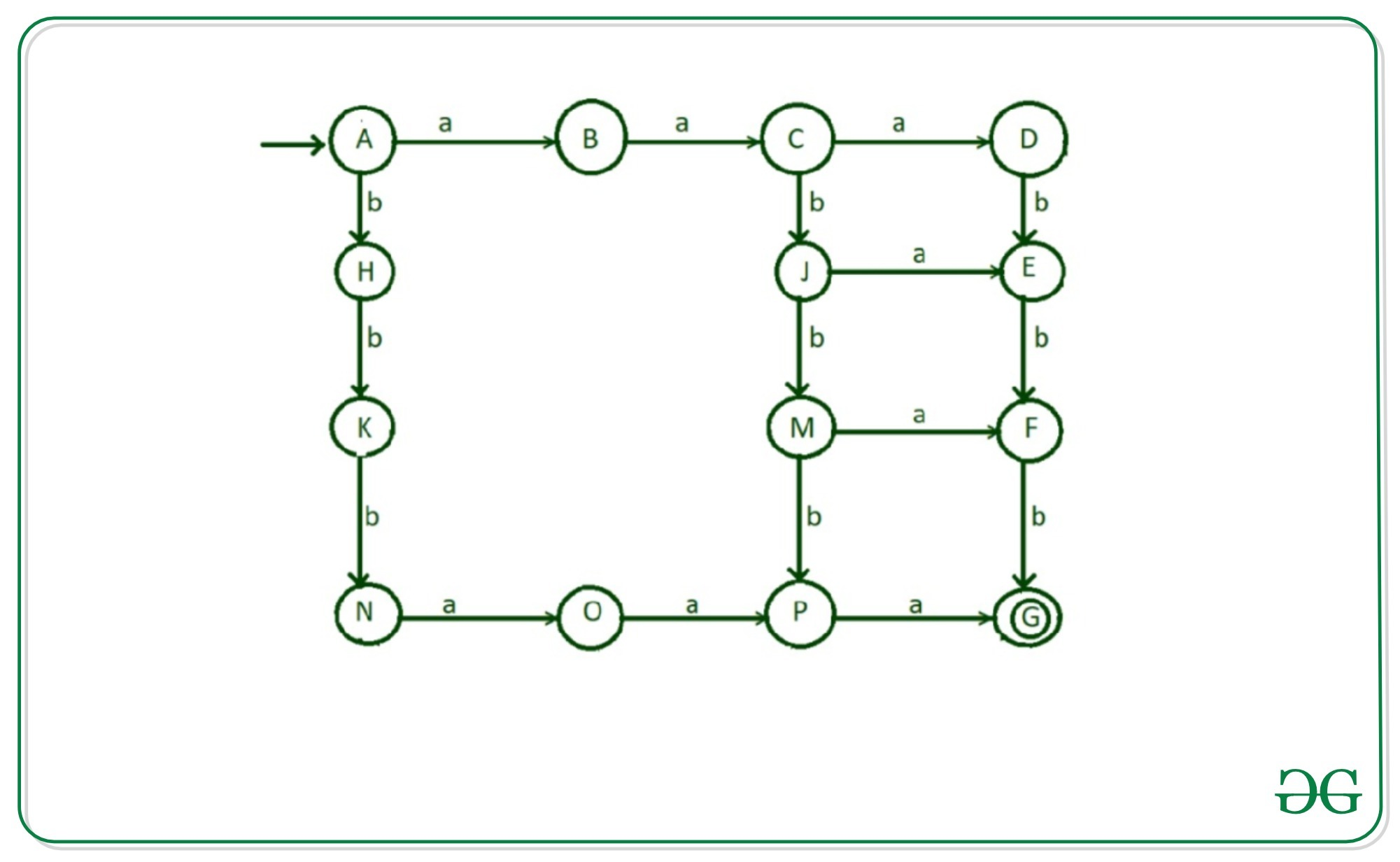Step-4: Now thinking of possibility that start with ‘aba’ and then fulfill the condition of given language.For this following transitions to be done:

• Transition of ‘b’ from state “B” to state “I”
• Transition of ‘a’ from state “I” to state “J”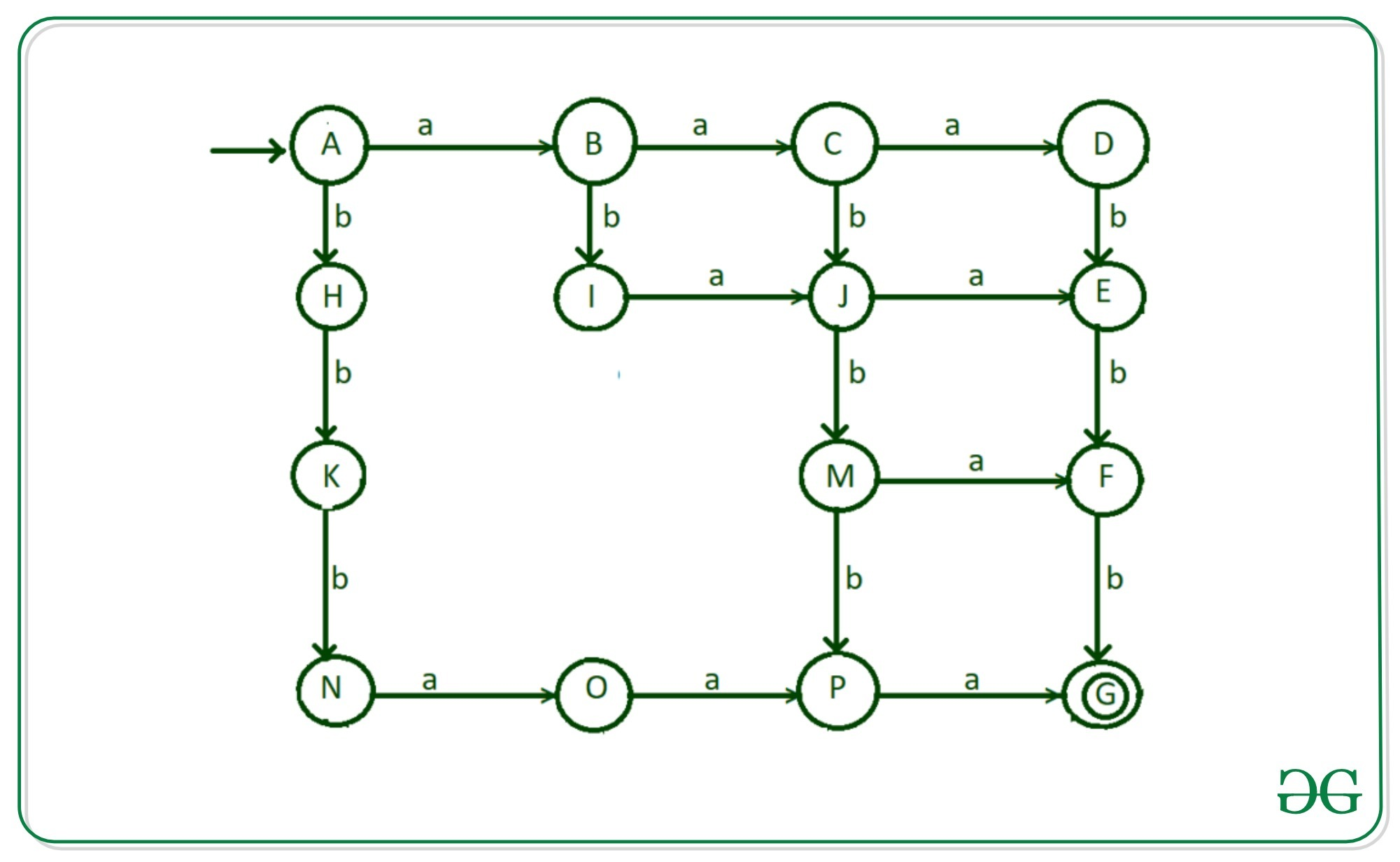Step-5: Now thinking of possibility that start with ‘abb’ and then fulfill the condition of given language. For this following transitions to be done:

• Transition of ‘b’ from state “I” to state “L”
• Transition of ‘a’ from state “L” to state “M”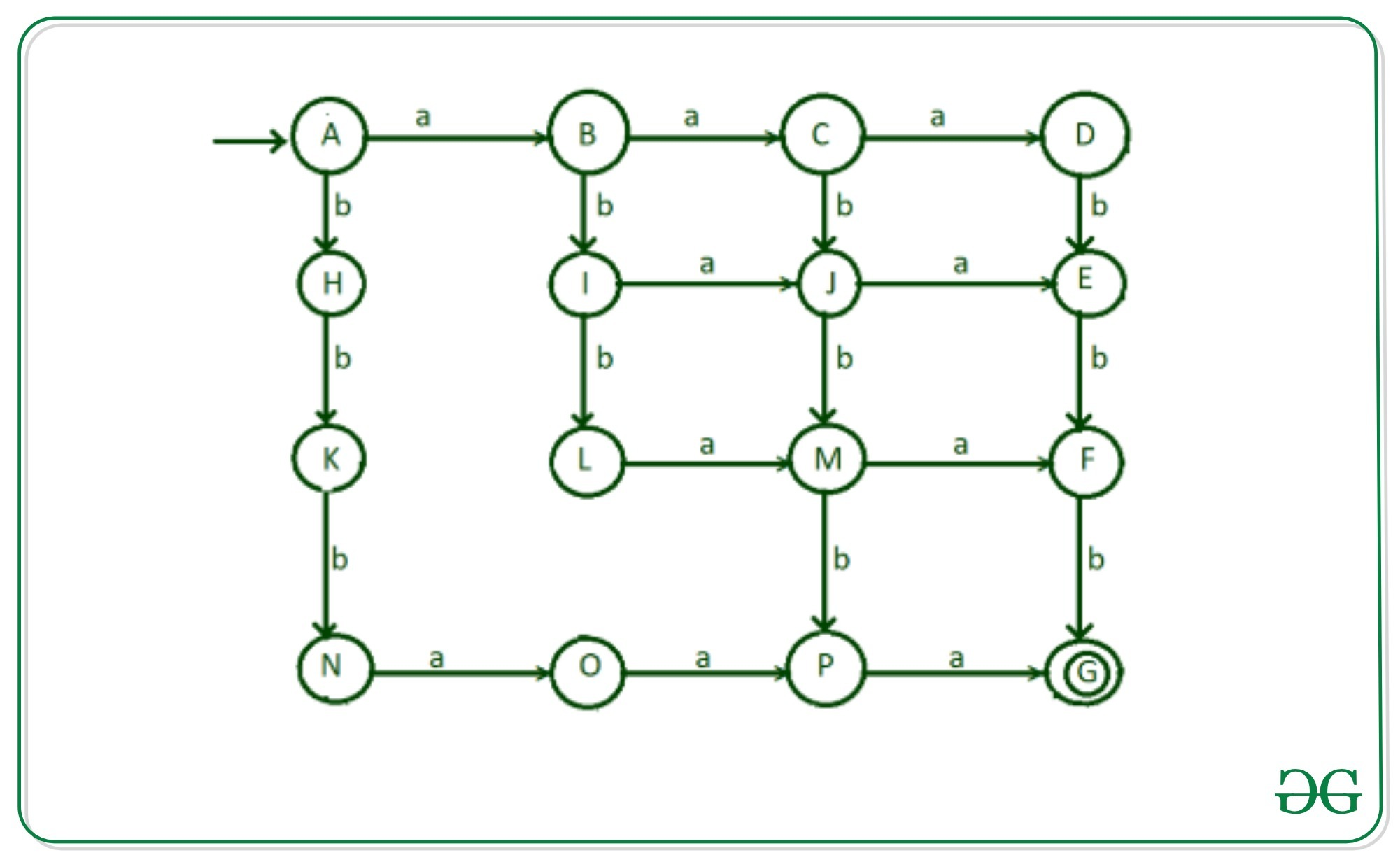Step-6: Now there is possibility that after reading one ‘a’ it reads all 3 b’s and then end execution by reading remaining 2 a’s.For transition of ‘b’ from state “L” to “O”.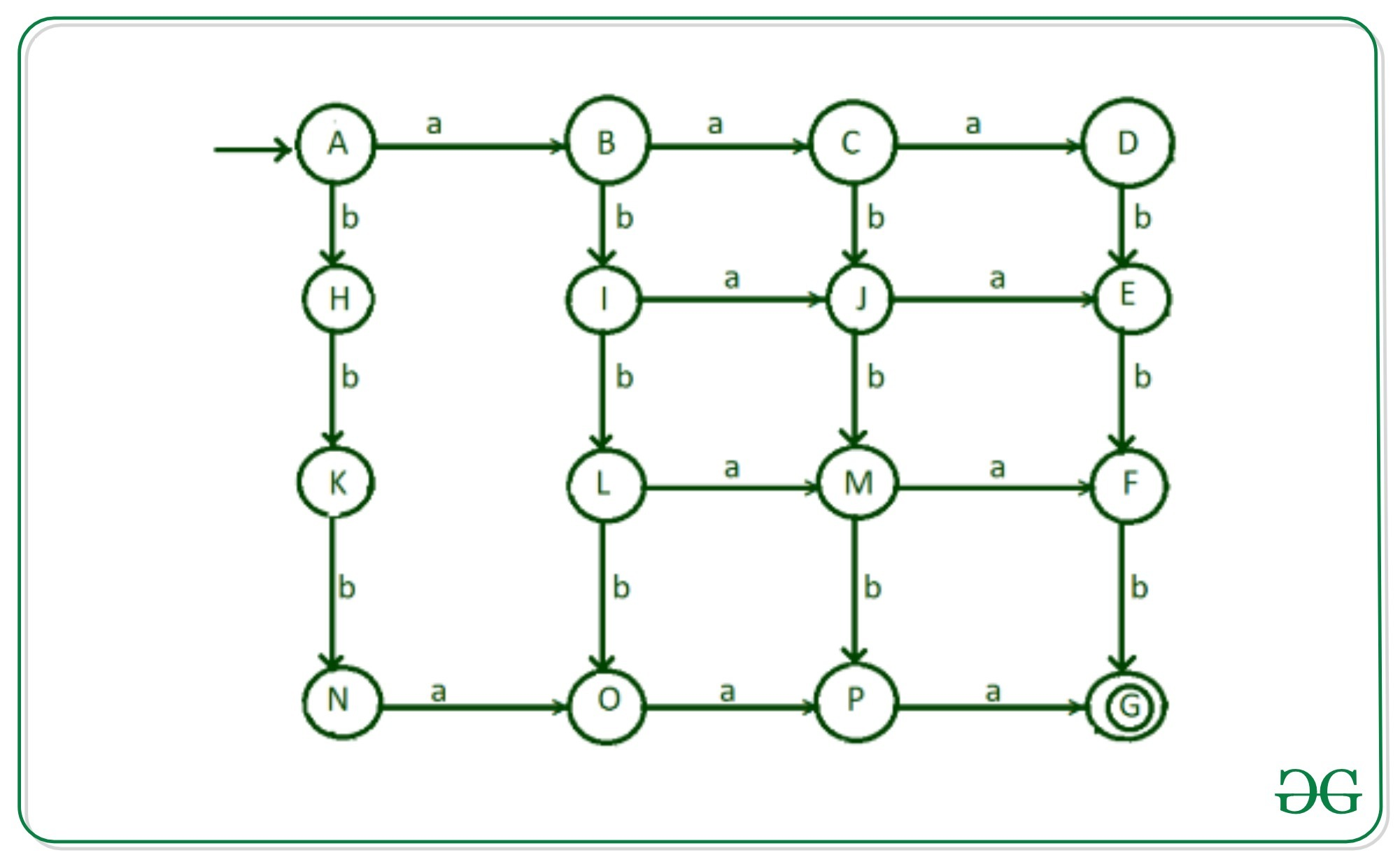Step-7: there is possibility that it reads ‘a’ after reading one ‘b’ and then it reads any combination of ‘a’ and ‘b’ so that string contains 3 a’s and 3 b’s.For this transition of ‘a’ is done from state”H” to state “I”.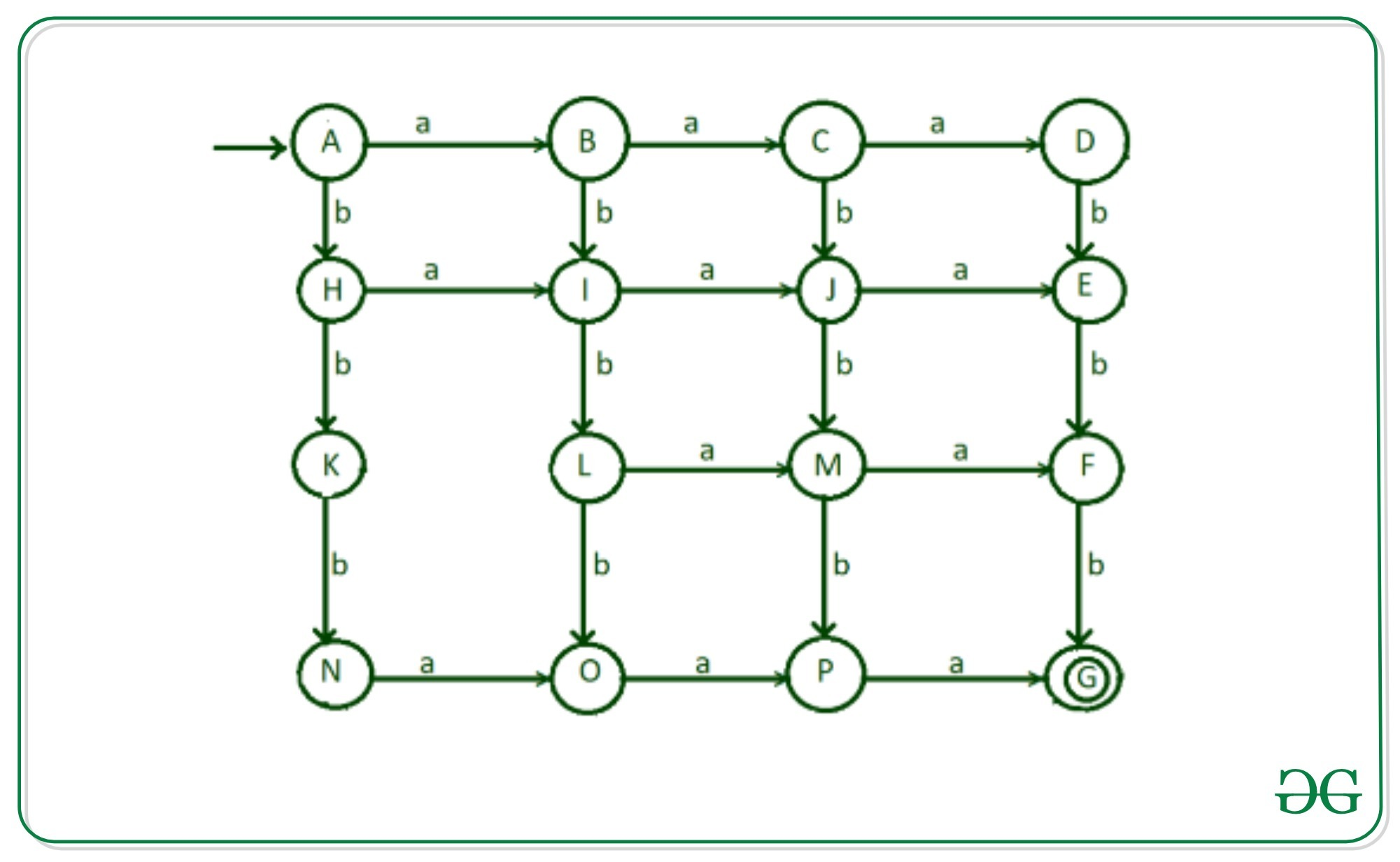Step-8: Now, think about the strings starts with two b’s and the go for any combination of string to get desired result for this we do transition of ‘a’ from stare “K” to state “L”.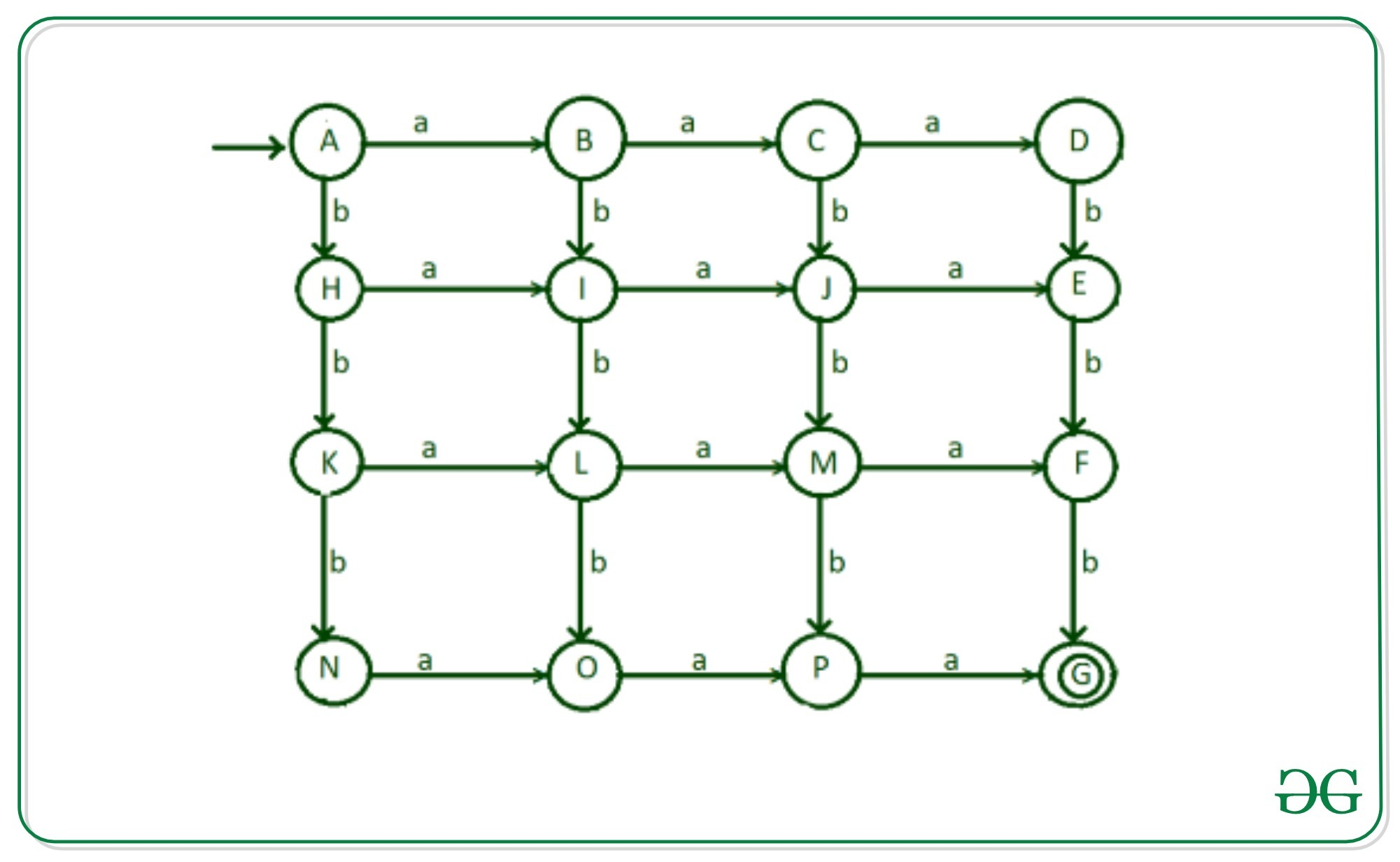Step-9: Now input alphabet ‘a’ of states D, E, F, G and ‘b’ of states G, N, O, P are left for transitions.AS we have done all possibilities of above language so remaining transition will go to dead states “Q1″ and Q2”.

• Transition of input ‘a’ from state “D” to dead state “Q1”
• Transition of input ‘a’ from state “E” to dead state “Q1”
• Transition of input ‘a’ from state “F” to dead state “Q1”
• Transition of input ‘a’ from state “G” to dead state “Q1”
• Transition of input ‘b’ from state “G” to dead state “Q2”
• Transition of input ‘b’ from state “N” to dead state “Q2”
• Transition of input ‘b’ from state “O” to dead state “Q2”
• Transition of input ‘b’ from state “P” to dead state “Q2”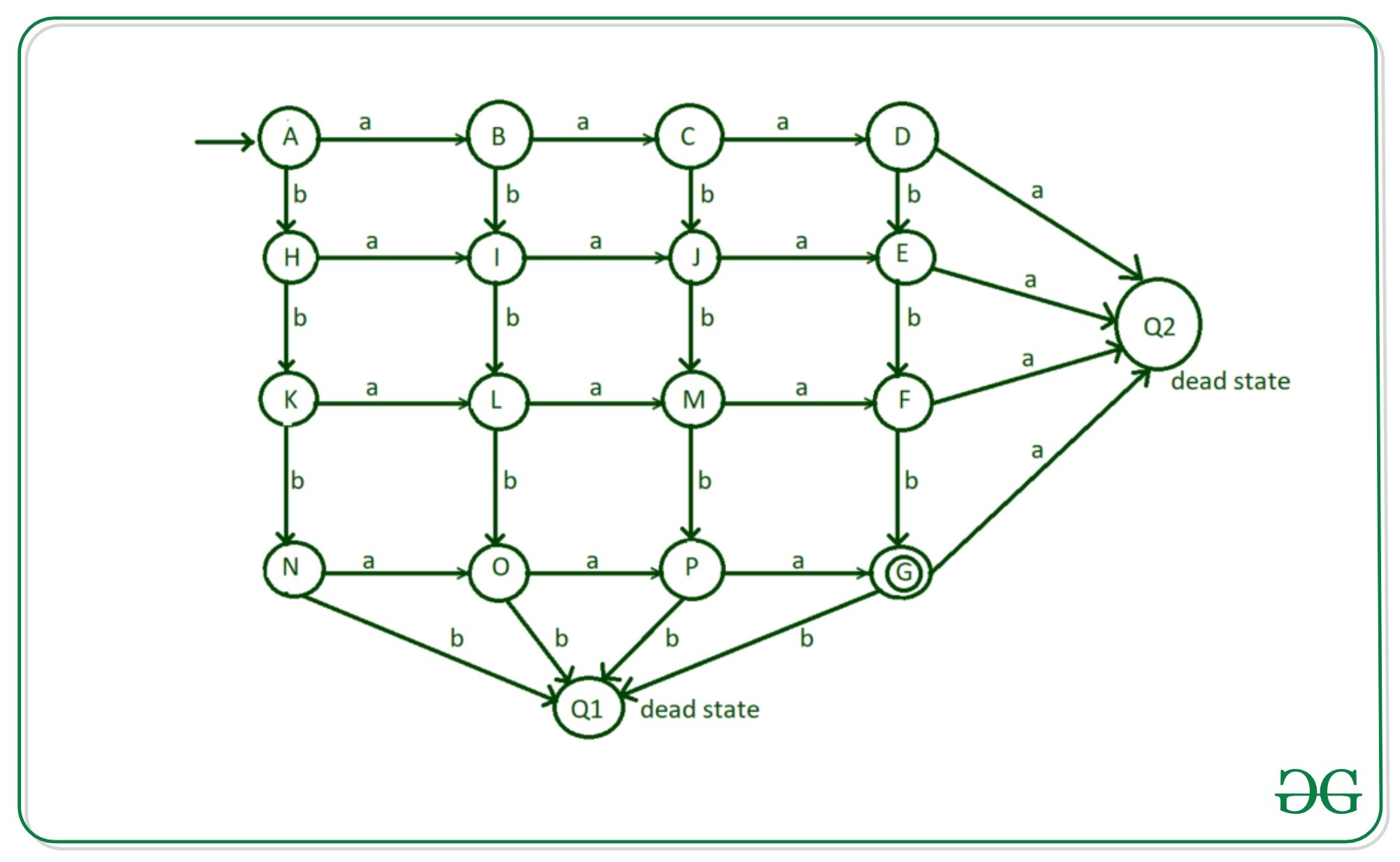Transition table of above DFA

STATES INPUT (a) INPUT (b)
—> A (initial state) B H
B C I
C D J
F Q2 (dead state) G*(final state)
H I K
I J L
J E M
K L N
L M O
M F P
P G* (final state) Q1 (dead state)

Below is the implementation of the DFA:

## Python3

 `# Python implementation of the  ` `# DFA of permutation of three  ` `# a's and three b's ` ` `  `# State A ` `def` `stateA(n): ` `    ``if``(n[``0``]``=``=``'a'``): ` `        ``stateB(n[``1``:]) ` `    ``elif` `(n[``0``]``=``=``'b'``): ` `        ``stateH(n[``1``:]) ` ` `  `# State B ` `def` `stateB(n): ` `    ``if``(``len``(n)``=``=` `0``): ` `        ``print``(``"String Not Accepted"``) ` `    ``else``:     ` `        ``if``(n[``0``]``=``=``'a'``): ` `            ``stateC(n[``1``:]) ` `        ``elif` `(n[``0``]``=``=``'b'``): ` `            ``stateI(n[``1``:]) ` ` `  `# State C ` `def` `stateC(n): ` `    ``if``(``len``(n)``=``=` `0``): ` `        ``print``(``"String Not Accepted"``) ` `    ``else``:     ` `        ``if``(n[``0``]``=``=``'a'``): ` `            ``stateD(n[``1``:]) ` `        ``elif` `(n[``0``]``=``=``'b'``): ` `            ``stateJ(n[``1``:]) ` ` `  `# State D ` `def` `stateD(n): ` `    ``if``(``len``(n)``=``=` `0``): ` `        ``print``(``"String Not Accepted"``) ` `    ``else``:     ` `        ``if``(n[``0``]``=``=``'a'``): ` `            ``stateQ2(n) ` `        ``elif` `(n[``0``]``=``=``'b'``): ` `            ``stateE(n[``1``:]) ` `         `  `# State E ` `def` `stateE(n): ` `    ``if``(``len``(n)``=``=` `0``): ` `        ``print``(``"String Not Accepted"``) ` `    ``else``:     ` `        ``if``(n[``0``]``=``=``'a'``): ` `            ``stateQ2(n) ` `        ``elif` `(n[``0``]``=``=``'b'``): ` `            ``stateF(n[``1``:])    ` `     `  `# State F ` `def` `stateF(n): ` `    ``if``(``len``(n)``=``=` `0``): ` `        ``print``(``"String Not Accepted"``) ` `    ``else``:     ` `        ``if``(n[``0``]``=``=``'a'``): ` `            ``stateQ2(n[``1``:]) ` `        ``elif` `(n[``0``]``=``=``'b'``): ` `            ``stateG(n[``1``:])      ` `            `  `# State G  ` `def` `stateG(n): ` `    ``if``(``len``(n)``=``=` `0``): ` `        ``print``(``"String Accepted"``) ` `    ``else``:     ` `        ``if``(n[``0``]``=``=``'a'``): ` `            ``stateQ2(n) ` `        ``elif` `(n[``0``]``=``=``'b'``): ` `            ``stateQ2(n)   ` `             `  `# State H ` `def` `stateH(n): ` `    ``if``(``len``(n)``=``=` `0``): ` `        ``print``(``"String Not Accepted"``) ` `    ``else``:     ` `        ``if``(n[``0``]``=``=``'a'``): ` `            ``stateI(n[``1``:]) ` `        ``elif` `(n[``0``]``=``=``'b'``): ` `            ``stateK(n[``1``:])  ` `            `  `# State I  ` `def` `stateI(n): ` `    ``if``(``len``(n)``=``=` `0``): ` `        ``print``(``"String Not Accepted"``) ` `    ``else``:     ` `        ``if``(n[``0``]``=``=``'a'``): ` `            ``stateJ(n[``1``:]) ` `        ``elif` `(n[``0``]``=``=``'b'``): ` `            ``stateL(n[``1``:])  ` ` `  `# State J ` `def` `stateJ(n): ` `    ``if``(``len``(n)``=``=` `0``): ` `        ``print``(``"String Not Accepted"``) ` `    ``else``:     ` `        ``if``(n[``0``]``=``=``'a'``): ` `            ``stateE(n[``1``:]) ` `        ``elif` `(n[``0``]``=``=``'b'``): ` `            ``stateM(n[``1``:])          ` `             `  `# State K ` `def` `stateK(n): ` `    ``if``(``len``(n)``=``=` `0``): ` `        ``print``(``"String Not Accepted"``) ` `    ``else``:     ` `        ``if``(n[``0``]``=``=``'a'``): ` `            ``stateL(n[``1``:]) ` `        ``elif` `(n[``0``]``=``=``'b'``): ` `            ``stateN(n[``1``:])   ` `             `  `# State L ` `def` `stateL(n): ` `    ``if``(``len``(n)``=``=` `0``): ` `        ``print``(``"String Not Accepted"``) ` `    ``else``:     ` `        ``if``(n[``0``]``=``=``'a'``): ` `            ``stateM(n[``1``:]) ` `        ``elif` `(n[``0``]``=``=``'b'``): ` `            ``stateO(n[``1``:])    ` `             `  `# State M ` `def` `stateM(n): ` `    ``if``(``len``(n)``=``=` `0``): ` `        ``print``(``"String Not Accepted"``) ` `    ``else``:     ` `        ``if``(n[``0``]``=``=``'a'``): ` `            ``stateF(n[``1``:]) ` `        ``elif` `(n[``0``]``=``=``'b'``): ` `            ``stateP(n[``1``:])             ` ` `  `# State N ` `def` `stateN(n): ` `    ``if``(``len``(n)``=``=` `0``): ` `        ``print``(``"String Not Accepted"``) ` `    ``else``:     ` `        ``if``(n[``0``]``=``=``'a'``): ` `            ``stateO(n[``1``:]) ` `        ``elif` `(n[``0``]``=``=``'b'``): ` `            ``stateQ1(n)  ` `             `  `# State Q ` `def` `stateO(n): ` `    ``if``(``len``(n)``=``=` `0``): ` `        ``print``(``"String Not Accepted"``) ` `    ``else``:     ` `        ``if``(n[``0``]``=``=``'a'``): ` `            ``stateP(n[``1``:]) ` `        ``elif` `(n[``0``]``=``=``'b'``): ` `            ``stateQ1(n)  ` `             `  `# State P ` `def` `stateP(n): ` `    ``if``(``len``(n)``=``=` `0``): ` `        ``print``(``"String Not Accepted"``) ` `    ``else``:     ` `        ``if``(n[``0``]``=``=``'a'``): ` `            ``stateG(n[``1``:]) ` `        ``elif` `(n[``0``]``=``=``'b'``): ` `            ``stateQ1(n[``1``:])      ` `             `  `# State Q1 ` `def` `stateQ1(n): ` `    ``print``(``"String Not Accepted"``) ` ` `  `# State Q2 ` `def` `stateQ2(n): ` `    ``print``(``"String Not Accepted"``) ` ` `  `# take string input ` `n ``=` `"abaabb"` ` `  `# call stateA ` `# to check the input ` `stateA(n) `

Output:

```String Accepted
```

Attention reader! Don’t stop learning now. Get hold of all the important CS Theory concepts for SDE interviews with the CS Theory Course at a student-friendly price and become industry ready.

My Personal Notes arrow_drop_upCheck out this Author's contributed articles.

If you like GeeksforGeeks and would like to contribute, you can also write an article using contribute.geeksforgeeks.org or mail your article to contribute@geeksforgeeks.org. See your article appearing on the GeeksforGeeks main page and help other Geeks.

Please Improve this article if you find anything incorrect by clicking on the "Improve Article" button below.